# Electroencephalography (EEG) based subject identification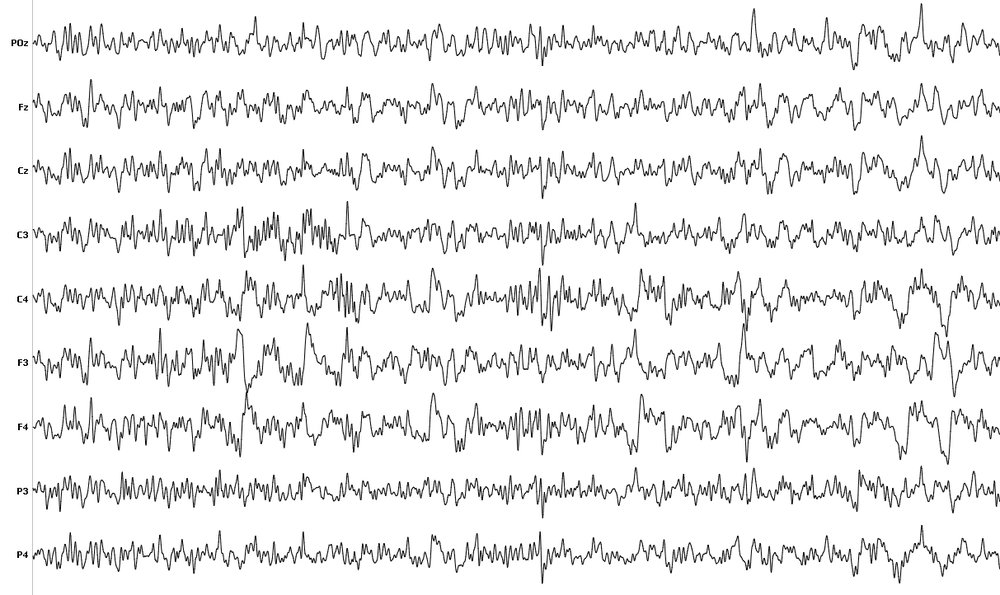## 1. Introduction

EEG is a non-invasive brain imaging technique that measures difference in electrical voltage (micro-voltage), that occur as a part of neural activity in the human brain. EEG signal data acquired from subjects are unique for every individual and offers stability in signal patterns that are needed in designing biometric systems and thus have been explored as a potential biometric trait.

A biometric system can perform either identification or authentication task. In identification, biometric system establishes the identity of the user i.e. it has a one-to-many (1: N) relationship while in authentication a biometric system verifies the claimed identity of the user (1:1) by matching the given sample with the stored template in the database. Here, we will perfrom identification of subjects' using publicly available dataset having EEG recordings from 48 subjects.

STEW-SI dataset consists of raw EEG data from 48 subjects who participated in a multitasking workload experiment utilizing the SIMKAP multitasking test. The subjects’ brain activity at rest was also recorded before the test and is included as well. The Emotiv EPOC device, with sampling frequency of 128Hz and 14 channels was used to obtain the data, with 2.5 minutes of EEG recording for each case. Subjects were also asked to rate their perceived mental workload after each stage on a rating scale of 1 to 9 and the ratings are provided in a separate file.

## 3. Methodology

Typical EEG subject identification pipeline includes tasks such as: signal-acquisition, signal pre-processing, features extraction, and classification phase.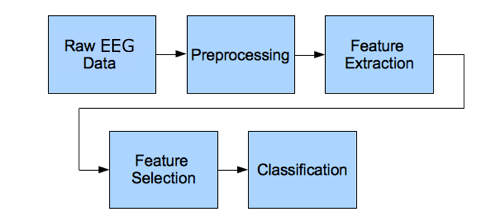Figure 1. EEG signal processing steps

Here, our EEG data is already in acquired form so, we can start from the next phase in the pipeline which is the signal pre-processing phase.

### 3.1 Signal pre-processing

Here we will filter our EEG dataset to remove noise and artifacts. For this purpose, we will use butterworth filter.

### Importing packages



import pandas as pd
import numpy as np
from numpy import mean
from numpy import std
import matplotlib.pyplot as plt
import scipy.io
import scipy.signal
from scipy.signal import butter,filtfilt,find_peaks,find_peaks, resample
from scipy import stats
from scipy.stats import skew, kurtosis
from sklearn import preprocessing
from sklearn.model_selection import train_test_split
from sklearn.metrics import classification_report,confusion_matrix,accuracy_score
from sklearn.metrics import roc_curve,auc, precision_score,recall_score,f1_score
from sklearn.feature_selection import SelectKBest, chi2
from sklearn.ensemble import ExtraTreesClassifier
from keras import layers, models, regularizers
import mne
from mne import find_events, fit_dipole
from autoreject import AutoReject
import seaborn as sns
import sys
import statistics as st
from keras.utils import to_categorical
#import pywt
import sys
import antropy as an
import time




Channels = ["AF3", "F7", "F3", "FC5", "T7", "P7", "O1", "O2", "P8", "T8", "FC6", "F4", "F8", "AF4"]

##1.1 Filter requirements.
T =     150         # Sample Period,    Seconds
fs =    128         # Sample rate,      Hz
cutoff = 40          # Desired cutoff frequency of the filter, Hz
nyq = 0.5 * fs      # Nyquist Frequency
order = 1           # sin wave can be approx represented as quadratic
n = int(T * fs)+1   # Total number of samples

t=np.linspace(0,150,19200)

normal_cutoff = [3/nyq ,40/nyq]
b, a = butter(order, normal_cutoff, btype='bandpass', analog=False)    # Get the filter coefficients
SS=0
TIMES = np.zeros()



### 3.2 Feature extraction

From the pre-processed EEG signal 17 features which included statistical, entropy, and energy features were extracted.



Features = ["mean_PSD",         "STD_PSD",
"A_mean",           "A_STD",            "A_Var",
"A_range",          "A_skew",           "A_kurtosis",
"Permutation_E",    "Spectral_E",       "SVD_E",
"Approximate_E",    "Sample_E",         "Petrosian_FD",
"Katz_FD",          "Higuchi_FD",
"Detrended fluctuation analysis",
"Label"]
# Features' Names
Features_df = pd.DataFrame(columns = Features)    # Features of Subject 1

start_time = time.time()
for s in range(1,49):
if s < 10:
URL=".\\STEW Dataset\\sub0"+str(s)+"_hi.txt"
else:
URL=".\\STEW Dataset\\sub"+str(s)+"_hi.txt"

Data.columns=Channels
print("Extracting Features From Subject: ",s)
print("--- %s seconds ---" % (time.time() - start_time))
TIMES[s-1] = time.time() - start_time

for Ch in Channels:
# Pre-Processing
Data.insert(0, ''.join([Ch + " Filtered"]), filtfilt(b,a,Data[Ch]))
Data = Data.drop(Ch, axis=1)
Data.insert(0, ''.join([Ch + " Despiked"]), Data[''.join([Ch + " Filtered"])].where(Data[''.join([Ch + " Filtered"])] < Data[''.join([Ch + " Filtered"])].quantile(0.97), Data[''.join([Ch + " Filtered"])].mean()))
Data = Data.drop(''.join([Ch + " Filtered"]), axis=1)
Data.insert(0, Ch, Data[''.join([Ch + " Despiked"])].where(Data[''.join([Ch + " Despiked"])] > Data[''.join([Ch + " Despiked"])].quantile(0.05), Data[''.join([Ch + " Despiked"])].mean()))
Data = Data.drop(''.join([Ch + " Despiked"]), axis=1)
w=0
for window in range(0,60):
EEG_Window = Data[w:w+640] # Windowing, Window Len: 5 Sec, Overlap: 2.5 Sec
w=w+320
Fet = np.zeros() #Temporal Features + Lable array
Fet_ch = np.zeros()
i=0
for i in range(14):
channel = EEG_Window[Channels[i]]
# Feature Extraction

# PSD Features
f, Pxx =scipy.signal.welch(channel,fs) #Extract PSD according to Welch thiorem

Fet[i]         = mean(Pxx)                                                      # Mean of PSD

Fet[i+14]       = std(Pxx)                                                      # Standered Deviation of PSD

# Statistics Features
Fet[i+28]      = mean(channel)                                                  # Amplitude Mean

Fet[i+42]      = std(channel)                                                   # Amplitude Standered Deviation

Fet[i+56]      = np.var(channel)                                                # Amplitude variance

Fet[i+70]      = max(channel)-min(channel)                                      # Amplitude Range

Fet[i+84]      = skew(channel)                                                  # Amplitude Skew

Fet[i+98]      = kurtosis(channel)                                              # Amplitude kurtosis

# Entropy Features
Fet[i+112]      = an.perm_entropy(channel, order=3, normalize=True)                 # Permutation entropy

Fet[i+126]      = an.spectral_entropy(channel, 100, method='welch', normalize=True) # Spectral entropy

Fet[i+140]      = an.svd_entropy(channel, order=3, delay=1, normalize=True)         # Singular value decomposition entropy

Fet[i+154]      = an.app_entropy(channel, order=2, metric='chebyshev')              # Approximate entropy

Fet[i+168]      = an.sample_entropy(channel, order=2, metric='chebyshev')           # Sample entropy

# Fractal dimension Features
Fet[i+182]      = an.petrosian_fd(channel)                                          # Petrosian fractal dimension

Fet[i+196]      = an.katz_fd(channel)                                               # Katz fractal dimension

Fet[i+210]      = an.higuchi_fd(channel, kmax=10)                                   # Higuchi fractal dimension

Fet[i+224]      = an.detrended_fluctuation(channel)                                 # Detrended fluctuation analysis

Fet_ch      = mean(Fet[0:14])        # Mean of PSD
Fet_ch      = mean(Fet[14:28])       # Standered Deviation of PSD
Fet_ch      = mean(Fet[28:42])       # Amplitude Mean
Fet_ch      = mean(Fet[42:56])       # Amplitude Standered Deviation
Fet_ch      = mean(Fet[56:70])       # Amplitude variance
Fet_ch      = mean(Fet[70:84])       # Amplitude Range
Fet_ch      = mean(Fet[84:98])       # Amplitude Skew
Fet_ch      = mean(Fet[98:112])      # Amplitude kurtosis
Fet_ch      = mean(Fet[112:126])     # Permutation entropy
Fet_ch      = mean(Fet[126:140])     # Spectral entropy
Fet_ch     = mean(Fet[140:154])     # Singular value decomposition entropy
Fet_ch     = mean(Fet[154:168])     # Approximate entropy
Fet_ch     = mean(Fet[168:182])     # Sample entropy
Fet_ch     = mean(Fet[182:196])     # Petrosian fractal dimension
Fet_ch     = mean(Fet[196:210])     # Katz fractal dimension
Fet_ch     = mean(Fet[210:224])     # Higuchi fractal dimension
Fet_ch     = mean(Fet[224:238])     # Detrended fluctuation analysis
Fet_ch     = s-1

Features_df.loc[SS]=Fet_ch
SS=SS+1
Features_df.to_csv('extractedFeatures.csv')
print("All features were extracted and saved")



Figure below shows the features map for the subject identification task.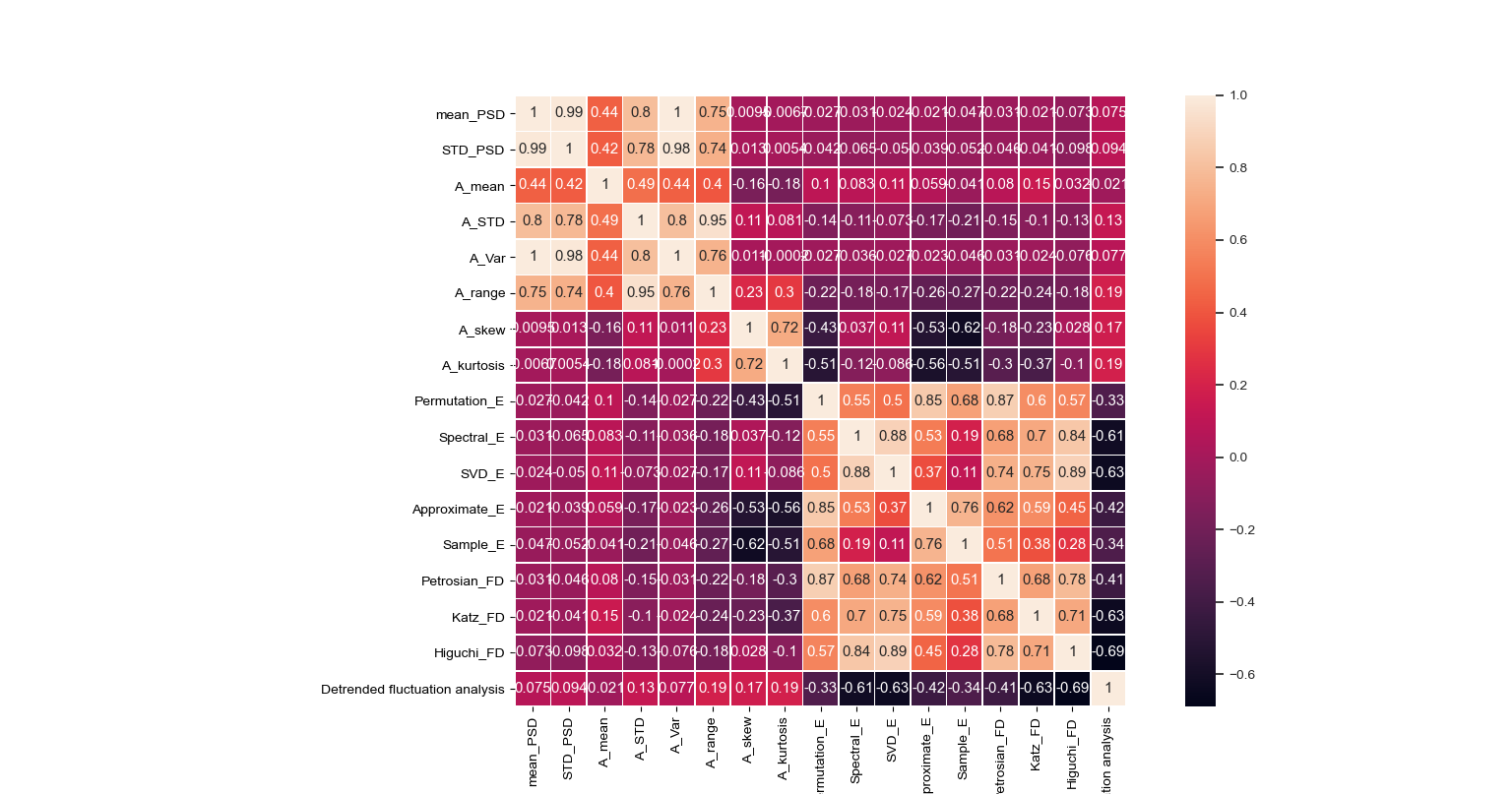Figure 2. Features Map.

### 3.3 Classification of subjects

For supplying data to the neural network model, we will label our data. Furthermore, we will split our labelled dataset into train, test, and validation sets.



## Shuffling
Data = Features_df.sample(frac = 1)
features = Data[[x for x in Data.columns if x not in ["Label"]]]   # Data for training
Labels = Data['Label']                                            # Labels for training
Labels = Labels.astype('category')

# Prepare Train and test Data
splitRatio = 0.3
train, test = train_test_split(Data ,test_size=splitRatio,
random_state = 123, shuffle = True)  # Spilt to training and testing data

train_X = train[[x for x in train.columns if x not in ["Label"]]]   # Data for training
train_Y = train['Label']                                            # Labels for training

###4.5.2 Testing Data
test_X = test[[x for x in test.columns if x not in ["Label"]]]     # Data fo testing
test_Y = test["Label"]                                              # Labels for training

###4.5.3 Validation Data
x_val = train_X[:200]                                                # 50 Sample for Validation
partial_x_train = train_X[200:]
partial_x_train = partial_x_train.values

y_val = train_Y[:200]
y_val = to_categorical(y_val)
partial_y_train = train_Y[200:]
partial_y_train = partial_y_train.values
partial_y_train = to_categorical(partial_y_train)

print("Data is prepeared")



#### 3.3.1 Building our Neural network classification model

For performing the identification task Artificial neural network (ANN) was used.



# Classification Model

## Building Model

### Architecture
model = models.Sequential()

### Hyper Parameters Tuning
loss='categorical_crossentropy',
metrics=['accuracy'])

print("Classifier Bulit\n")
print("Start Training\n")

## Training Model
history = model.fit(partial_x_train,
partial_y_train,
epochs = 5,                # initial value = 1000
batch_size = 16,              # initial value = 16
validation_data=(x_val, y_val))

weights = model.get_weights()
configs = model.get_config()

Featuers_weights = np.apply_along_axis(mean, 1, weights)
print("Finish Training")



#### 3.3.2 Model Evaluation

Here, we will evaluate our build NN model performance in terms of training-validation accuracy and train-validation loss.



## Network Architecture
print(model.summary())
print("Start Evaluating Data")

## Training Process
history_dict = history.history
loss_values = history_dict['loss']
val_loss_values = history_dict['val_loss']

epochs = range(1,len(loss_values)+1)
plt.figure()
plt.plot(epochs, loss_values, 'bo', label="training loss", color='r')
plt.plot(epochs, val_loss_values, 'b', label="validation loss")
plt.title("Training and Validation Loss")
plt.xlabel("Epoches")
plt.ylabel("loss")
plt.legend()
plt.show()

acc_values = history_dict['accuracy']
val_acc_values = history_dict['val_accuracy']
plt.figure()
plt.plot(epochs, acc_values, 'bo', label="training acc", color = 'r')
plt.plot(epochs, val_acc_values, 'b', label="validation acc")
plt.title("Training and Validation acc")
plt.xlabel("Epoches")
plt.ylabel("acc")
plt.legend()
plt.show()



Figures below shows the training-validation loss and training-validation accuracy curves.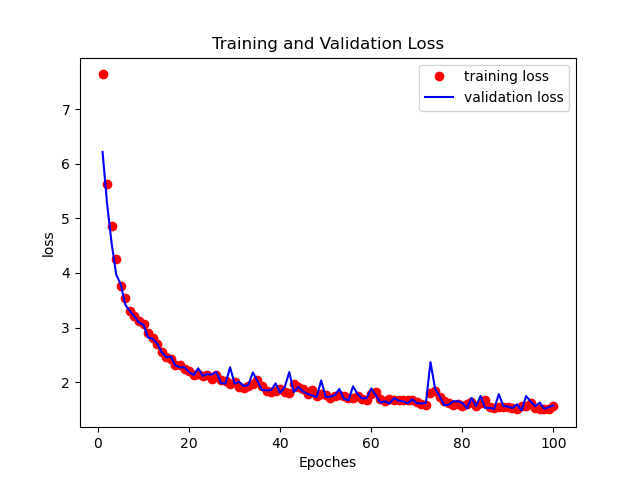Figure 3. Training-validation loss curve.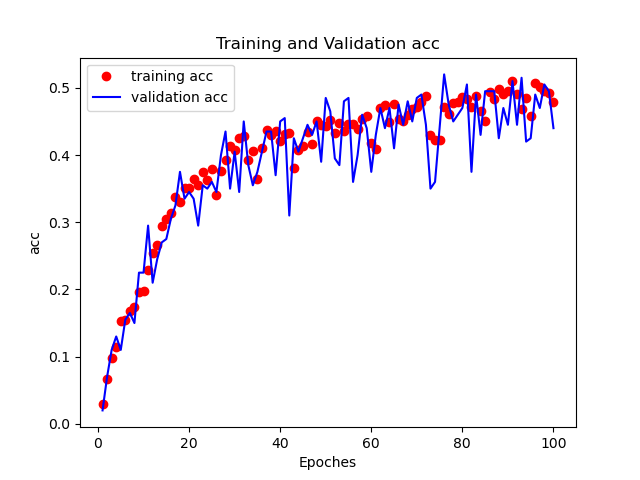Figure 4. Training-validation accuracy curve.

#### 3.3.3 Neural Network model evaluation on test data

Evaluating our NN model on test data and making predictions.



# Model Evaluation on Test data
## Prediction
ANN_predictions = model.predict(test_X)

Pred = np.zeros([len(ANN_predictions)])
for i in range(0,len(ANN_predictions)):
Pred[i] = list(ANN_predictions[i]).index(max(ANN_predictions[i]))
ANN_Pred = pd.Series(Pred)



#### 3.3.4 Results

For the evaluation of the performance of our NN classification model we will use standard metrics and plot the confusion matrix for the same.



#### Metrics
print("Accuracy:",accuracy_score(test_Y, ANN_Pred))
print("f1 score:", f1_score(test_Y, ANN_Pred,average="micro"))
print("precision score:", precision_score(test_Y, ANN_Pred,average="micro"))
print("recall score:", recall_score(test_Y, ANN_Pred,average="micro"))
print("confusion matrix:\n",confusion_matrix(test_Y, ANN_Pred))
print("classification report:\n", classification_report(test_Y, ANN_Pred))

#### Plots

# plot Confusion Matrix as heat map
plt.figure(figsize=(3,2))
sns.heatmap(confusion_matrix(test_Y, ANN_Pred),annot=True,fmt = "d",linecolor="k",linewidths=3)
plt.title("CONFUSION MATRIX",fontsize=20)
plt.show()


Figure below shows the confusion matrix for the subject identification task.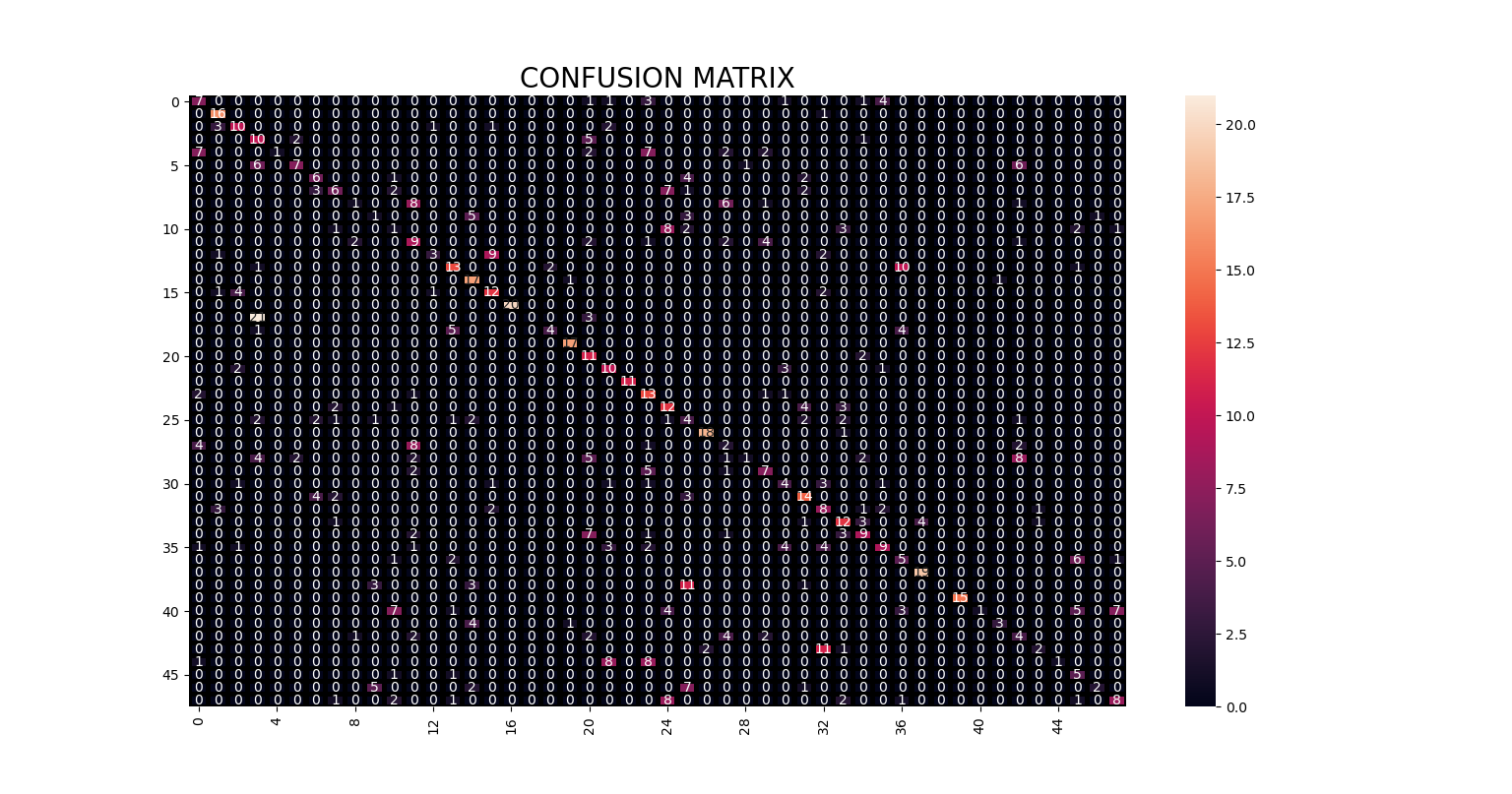Figure 5. Confusion matrix for the subject identification task.

## 4. Conclusion

In this article, we build and tested our Neural Network model for the subject identification task on EEG data from 48 subjects. Our proposed model achieved an accuracy of 54% in correctly predicting subjects’ identity using their brain signals. Less accuracy may be attributed to the fewer data present for training and testing purpose.

Complete code can be found here.

• Khan, T. A., & Jabin, S. (2021). Recent trends in electroencephalography based biometric systems. In Recent Trends in Communication and Electronics (pp. 467-472). CRC Press.
• Wei Lun Lim, Olga Sourina, Lipo Wang, July 10, 2018, "STEW: Simultaneous Task EEG Workload Dataset", IEEE Dataport, doi: https://dx.doi.org/10.21227/44r8-ya50.